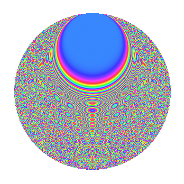# Properties

 Label 2541.2.sLevel 2541 Weight 2 Character orbit s Rep. character $$\chi_{2541}(239,\cdot)$$ Character field $$\Q(\zeta_{10})$$ Dimension 864 Sturm bound 704

# Related objects

## Defining parameters

 Level: $$N$$ = $$2541 = 3 \cdot 7 \cdot 11^{2}$$ Weight: $$k$$ = $$2$$ Character orbit: $$[\chi]$$ = 2541.s (of order $$10$$ and degree $$4$$) Character conductor: $$\operatorname{cond}(\chi)$$ = $$33$$ Character field: $$\Q(\zeta_{10})$$ Sturm bound: $$704$$

## Dimensions

The following table gives the dimensions of various subspaces of $$M_{2}(2541, [\chi])$$.

Total New Old
Modular forms 1504 864 640
Cusp forms 1312 864 448
Eisenstein series 192 0 192

## Trace form

 $$864q - 8q^{3} - 216q^{4} + 10q^{6} + 22q^{9} + O(q^{10})$$ $$864q - 8q^{3} - 216q^{4} + 10q^{6} + 22q^{9} + 52q^{12} - 6q^{15} - 208q^{16} + 10q^{18} + 60q^{19} - 30q^{24} + 240q^{25} - 8q^{27} + 40q^{28} - 60q^{30} - 52q^{31} - 8q^{34} + 6q^{36} - 28q^{37} + 10q^{39} + 168q^{45} + 40q^{46} - 68q^{48} + 216q^{49} + 30q^{51} + 40q^{52} + 30q^{57} - 24q^{58} + 20q^{60} - 40q^{61} - 224q^{64} + 104q^{67} - 16q^{69} - 32q^{70} - 150q^{72} - 40q^{73} - 2q^{75} + 52q^{78} + 40q^{79} - 6q^{81} + 16q^{82} - 40q^{84} + 60q^{85} - 100q^{90} - 36q^{91} + 50q^{93} - 160q^{96} + 100q^{97} + O(q^{100})$$

## Decomposition of $$S_{2}^{\mathrm{new}}(2541, [\chi])$$ into newform subspaces

The newforms in this space have not yet been added to the LMFDB.

## Decomposition of $$S_{2}^{\mathrm{old}}(2541, [\chi])$$ into lower level spaces

$$S_{2}^{\mathrm{old}}(2541, [\chi]) \cong$$ $$S_{2}^{\mathrm{new}}(33, [\chi])$$$$^{\oplus 4}$$$$\oplus$$$$S_{2}^{\mathrm{new}}(231, [\chi])$$$$^{\oplus 2}$$$$\oplus$$$$S_{2}^{\mathrm{new}}(363, [\chi])$$$$^{\oplus 2}$$

## Hecke Characteristic Polynomials

There are no characteristic polynomials of Hecke operators in the database